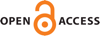Download this articleFor screen For printingRecent IssuesThe Journal About the Journal Editorial Board Editorial Interests Subscriptions Submission Guidelines Submission Page Policies for Authors Ethics Statement ISSN (electronic): 1472-2739 ISSN (print): 1472-2747 Author Index To Appear Other MSP JournalsRealization of graded monomial ideal rings modulo torsion

### Tseleung So and Donald Stanley

Algebraic & Geometric Topology 23 (2023) 733–764##### Abstract

Let $A$ be the quotient of a graded polynomial ring $ℤ\left[{x}_{1},\dots ,{x}_{m}\right]\otimes \Lambda \left[{y}_{1},\dots ,{y}_{n}\right]$ by an ideal generated by monomials with leading coefficients $1$. We construct a space ${X}_{A}$ such that $A$ is isomorphic to ${H}^{\ast }\left({X}_{A}\right)$ modulo torsion elements.

##### Keywords
cohomology realization problem, polyhedral product
##### Mathematical Subject Classification
Primary: 55N10
Secondary: 13F55, 55P99, 55T20
##### Publication